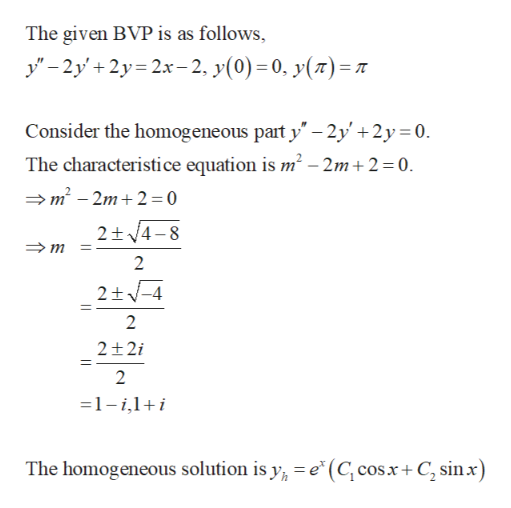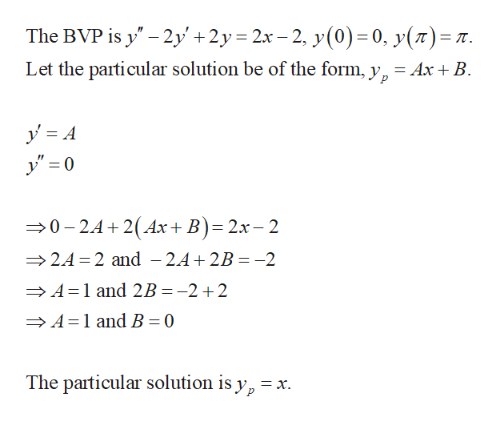# Solve the given boundary-value problem.y'' − 2y' + 2y = 2x − 2,  y(0) = 0, y(π) = π

Question
232 views
Solve the given boundary-value problem.
y'' − 2y' + 2y = 2x − 2,  y(0) = 0, y(π) = π
check_circle

Step 1

Find the homogeneous solution of the given IVP as follows.help_outlineImage TranscriptioncloseThe given BVP is as follows, -2y2y2x-2, y(0)= 0, y(z)=7 Consider the homogeneous part y 2y'+2y 0 The characteristi ce equation is m2 - 2m+ 2 0. m2 - 2m+2 = 0 2+/4-8 2 2+-4 2 2+2i 2 1- i,1+ i The homogeneous solution is y e* (C cosx+ C2 sinx) fullscreen
Step 2

Find the particular solution of ...help_outlineImage TranscriptioncloseThe BVP is y" 2y'+2y 2x-2, y(0) = 0, y(7)=T. Let the particular solution be of the form, y, = Ax +B 02.4 2(Ax+ B)= 2x- 2 24 2 and -24+2B -2 A 1 and 2B =-2+2 A 1 and B = 0 The particular solution is yp = = x. fullscreen

### Want to see the full answer?

See Solution

#### Want to see this answer and more?

Solutions are written by subject experts who are available 24/7. Questions are typically answered within 1 hour.*

See Solution
*Response times may vary by subject and question.
Tagged in

### Math